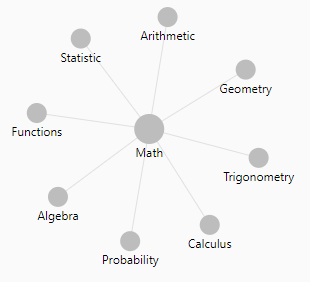$b^2$ is usually read as b squared.

Squared is usually used in formula to get rid of the sign. To go back on the same scale, the root squared operator is used.

## Inverse

The inverse function of square is the square root.

Recommended PagesMathematics - Exponentiation (square, cube) - Power

Exponentiation is a binary operation involving two numbers: the base (b) the exponent (n) (or index or power). In text notation or computer language, generally the exponentiation operator...Mathematics - Quadratic (function|polynomial of degree 2)

In mathematics, a quadratic function, a quadratic polynomial, a polynomial of degree 2, or simply a quadratic, is a polynomial function in one or more variables in which the highest-degree...Number - Root function

The root function is the inverse operation of the exponentiation. With the below exponentiation of the base b to the power of n the base b can be defined back with the root function as: nlogarithm...